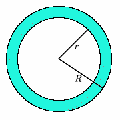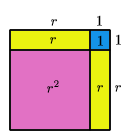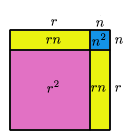#### You may also like### Algebra Match

A task which depends on members of the group noticing the needs of others and responding.### Simplifying Doughnut

An algebra task which depends on members of the group noticing the needs of others and responding.

# Algebra from Geometry

##### Age 11 to 16Challenge Level

Michael Brooker tackled the problem Bull's Eye and noticed something interesting when finding the area of a ring, which he has followed up. The result he found will be familiar to many older mathematicians.Michael could see that the formula for the area of a ring must be pi(R 2 - r 2 ). What he noticed was that in the examples he worked out, the area also appeared to be pi(R+r). This interested him, and he realised that you can find the difference between numbers with consecutive square roots by adding the roots:
144 - 121 = 23 = 12 + 11

Michael realised that this result is the reason why the second formula works for rings where R = r + 1, and tried to prove why.
He used a geometrical method to show that (r + 1) 2 = r 2 + 2r + 1:So, if R = r + 1,
R 2 - r 2 = (r 2 + 2r + 1) - r 2 = 2r + 1 = R + r

We asked Michael what happened if the ring was 2 wide, or 3 wide. Could he find an alternative formula for these rings, similar to the simple one he found above? He started by trying some examples, to see if he could spot anything interesting.

r R area width R+r
2 4 12pi 2 6
2 5 21pi 3 7
2 6 32pi 4 8

Have you spotted what Michael did?

He then set out to prove that the formula for the area of a ring is pi(R - r)(R + r)

Michael ignored the pi, and tried to show that
R 2 - r 2 = (R - r)(R + r).
This result is known as "the difference of two squares". It is a very useful result, and Michael did well to find out about it for himself.

He introduced the letter n for the width, so R = r + n, and then rewrote the formulas above using r and n instead of r and R.

(R - r)(R + r) = n(r + n + r) = n(2r + n)
R 2 - r 2 = (r + n) 2 - r 2 = (r + n)(r + n) - r 2

Even if you don't know how to do (r + n)(r + n), you can probably see what it is from the diagram below.So (r + n)(r + n) - r 2 = r 2 + rn + rn + n 2 - r 2 = 2rn + n 2 = n(2r + n)

So R 2 - r 2 = (R - r)(R + r), and Michael has proved the result. Well done!

You may like to look at a couple of problems which are based on the the "Difference Of Two Squares" result: What's Possible? and Plus Minus